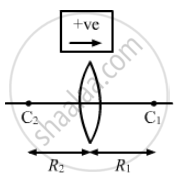Advertisement Remove all ads

# A Double Convex Lens Has Focal Length 25 Cm. the Radius of Curvature of One of the Surfaces is Double of - Science

Sum

A double convex lens has focal length 25 cm. The radius of curvature of one of the surfaces is double of the other. Find the radii, if the refractive index of the material of the lens is 1.5.

Advertisement Remove all ads

#### Solution

Given,
Double convex lens of focal length, f = 25 cm
Refractive index of the material, μ = 1.5
As per the question, the radius of curvature of one surface is twice that of the other.
i.e. R1= R and R2= −2R
(according to sign conventions)

Using the lens maker formula
$\frac{1}{f} = (\mu - 1)\left[ \frac{1}{R_1} - \frac{1}{R_2} \right]$' we have:
$\frac{1}{25} = \left( 1 . 5 - 1 \right)\left[ \frac{1}{R} - \left( \frac{1}{- 2R} \right) \right]$

$\Rightarrow \frac{1}{25} = 0 . 5\left[ \frac{3R}{2} \right]$

$\Rightarrow \frac{1}{25} = \left[ \frac{3R}{4} \right]$

$\Rightarrow R = 18 . 75 \text{ cm }$

R1 = 18.75 cm and R2 = 37.5 cm

Hence, the required radii of the double convex lens are 18.75 cm and 37.5 cm.Is there an error in this question or solution?
Advertisement Remove all ads

#### APPEARS IN

HC Verma Class 11, 12 Concepts of Physics 1
Chapter 18 Geometrical Optics
Q 48 | Page 415
Advertisement Remove all ads
Advertisement Remove all ads
Share
Notifications

View all notifications

Forgot password?
Course